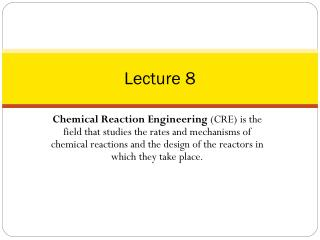Download PresentationLecture 8

Loading in 2 Seconds...

# Lecture 8 - PowerPoint PPT Presentation

Lecture 8. Chemical Reaction Engineering (CRE) is the field that studies the rates and mechanisms of chemical reactions and the design of the reactors in which they take place. Lecture 8 – Tuesday 2/5/2013. Block 1: Mole Balances Block 2: Rate Laws Block 3: StoichiometryI am the owner, or an agent authorized to act on behalf of the owner, of the copyrighted work described.
Download Presentation## Lecture 8

An Image/Link below is provided (as is) to download presentation

Download Policy: Content on the Website is provided to you AS IS for your information and personal use and may not be sold / licensed / shared on other websites without getting consent from its author.While downloading, if for some reason you are not able to download a presentation, the publisher may have deleted the file from their server.

- - - - - - - - - - - - - - - - - - - - - - - - - - E N D - - - - - - - - - - - - - - - - - - - - - - - - - -
Presentation Transcript
1. Lecture 8 Chemical Reaction Engineering (CRE) is the field that studies the rates and mechanisms of chemical reactions and the design of the reactors in which they take place.

2. Lecture 8 – Tuesday 2/5/2013 • Block 1: Mole Balances • Block 2: Rate Laws • Block 3: Stoichiometry • Block 4: Combine • Pressure Drop • Liquid Phase Reactions • Gas Phase Reactions • Engineering Analysis of Pressure Drop

3. Pressure Drop in PBRs Concentration Flow System: Gas Phase Flow System:

4. Pressure Drop in PBRs Note: Pressure Drop does NOT affect liquid phase reactions Sample Question: Analyze the following second order gas phase reaction that occurs isothermally in a PBR: AB Mole Balances Must use the differential form of the mole balance to separate variables: Rate Laws Second order in A and irreversible:

5. Pressure Drop in PBRs Stoichiometry Isothermal, T=T0 Combine: Need to find (P/P0) as a function of W (or V if you have a PFR)

6. Pressure Drop in PBRs ErgunEquation: Constant mass flow:

7. Pressure Drop in PBRs Variable Density Let

8. Pressure Drop in PBRs Where CatalystWeight Let

9. Pressure Drop in PBRs Wewilluse this form for singlereactions: Isothermal case

10. Pressure Drop in PBRs and or The two expressions are coupledordinary differential equations. Wecanonlysolvethemsimultaneouslyusing an ODE solversuch as Polymath. For the special case of isothermal operation and epsilon = 0, we can obtain an analytical solution. Polymath will combine the Mole Balances, Rate Laws and Stoichiometry.

11. Packed Bed Reactors

12. Reaction Rate in a PBR 3 -rA W

13. Conversion in a PBR 4 X W

14. Flow Rate in a PBR 5 1 W

15. Example 1:Gas Phase Reaction in PBR for δ=0 Gas Phase reaction in PBR with δ = 0 (Analytical Solution) A + B  2C Repeat the previous one with equimolar feed of A and B and: kA = 1.5dm6/mol/kg/min α = 0.0099 kg-1 Find X at 100 kg

16. Example 1:Gas Phase Reaction in PBR for δ=0 1) Mole Balance 2) Rate Law 3) Stoichiometry

17. Example 1:Gas Phase Reaction in PBR for δ=0 , , 4) Combine

18. Example 1:Gas Phase Reaction in PBR for δ=0

19. Example 2:Gas Phase Reaction in PBR for δ≠0 The reaction A + 2B  C is carried out in a packed bed reactor in which there is pressure drop. The feed is stoichiometric in A and B. Plot the conversion and pressure ratio y = P/P0 as a function of catalyst weight up to 100 kg. Additional Information kA = 6 dm9/mol2/kg/min α = 0.02 kg-1

20. Example 2:Gas Phase Reaction in PBR for δ≠0 A + 2B  C 3) Stoichiometry: Gas, Isothermal 1) Mole Balance 2) Rate Law

21. Example 2:Gas Phase Reaction in PBR for δ≠0 4) 7) 6) 5) Initial values: W=0, X=0, y=1 Final values: W=100 Combine with Polymath. If δ≠0, polymath must be used to solve.

22. Example 2:Gas Phase Reaction in PBR for δ≠0

23. Example 2:Gas Phase Reaction in PBR for δ≠0

24. T = T0

25. Robert the Worrier wonders: What if we increase the catalyst size by a factor of 2?

26. Pressure DropEngineering Analysis

27. Pressure DropEngineering Analysis

28. Pressure DropEngineering Analysis

29. Heat Effects Isothermal Design Stoichiometry Rate Laws Mole Balance

30. End of Lecture 8

31. Pressure Drop - Summary • Pressure Drop • Liquid Phase Reactions • Pressure Drop does not affect concentrations in lquid phase reactions. • Gas Phase Reactions • Epsilon does not equal to zero d(P)/d(W)=… Polymath will combine with d(X)/d(W) =… for you • Epsilon = 0 and isothermal P=f(W) Combine then separate variables (X,W) and integrate • Engineering Analysis of Pressure Drop

32. Pressure Change – Molar Flow Rate Use for heat effects, multiple rxns Isothermal: T = T0

33. Example 1:Gas Phase Reaction in PBR for δ=0 A + B  2C Case 1: Case 2:

34. PBR